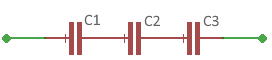# Calculate capacitor series connection

Calculators and formulas for calculating a capacitor series connection

## Capacitor series connection

When capacitors are connected in series, the total current flows through all capacitors.## Calculation of the series connection of capacitors

To calculate the capacitance, enter the values of the individual capacitors separated by a seccolon.
Example: 3.3; 12; 22

Exponents are not allowed. Instead, enter the values in a suitable unit of measure. If you enter all values in Nano-Farad, the result is also displayed in Nano-Farad.

Capacitor series calculator

 Enter the capacitors separated by a semicolon Result Decimal places 0 1 2 3 4 6 8 10

## Formulas to calculate the total capacity

The total capacitance of a series connection is calculated using the reciprocal of the individual capacitors. The individual reciprocal values are added to calculate the total capacity. So the formula for three capacitors connected in series is:

$$\displaystyle \frac{1}{C_{ges}}=\frac{1}{C_1}+\frac{1}{C_2}+\frac{1}{C_3}$$

The following formula can be used to calculate the total capacitance of two capacitors connected in series.

$$\displaystyle C_{ges}=\frac{C_1· C_2}{C_1 + C_2}$$